# High School Physics : Interference and Superposition

## Example Questions

### Example Question #59 : Waves

The siren of a police car at rest emits a frequency of about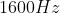.  What frequency will you hear if you are at rest and the police car moves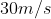away from you?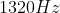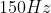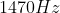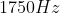Explanation:

This is an example of the doppler effect.  In this case the source is moving away from a stationary observer.  Therefore the corresponding equation is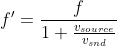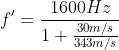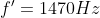### Example Question #60 : Waves

Two waves, one with an amplitude of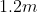and the other of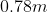are superimposed with destructive interference. What is the resultant amplitude?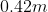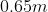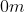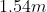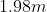Explanation:

When two waves are superimposed, the interference can be either constructive or destructive. In this case the interference is destructive, which means our resultant amplitude will be the difference of the two given amplitudes.

That means our new amplitude will be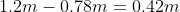.

### Example Question #981 : High School Physics

A piano tuner hears one beat every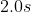when trying to adjust two strings, one of which is sounding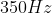.  How far off in frequency is the other string?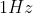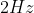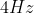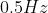Explanation:

We can calculate the frequency of the beat using the equation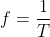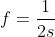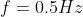This is the beat frequency which means that the other string is off byeither higher or lower.

### Example Question #982 : High School Physics

Resonance in a system, such as a string fixed on both ends, occurs when

It is oscillating in simple harmonic motion

Its frequency is smaller than the frequency of an external source

Its frequency is greater than the frequency of an external source

Its frequency is the same as the frequency of an external source

Its frequency is the same as the frequency of an external source

Explanation:

When we think about a violin or a guitar string that is being plucked, there are a variety of waves of different frequencies that will travel along the string and be reflected back at the ends.  Most interfere with each other and disappear.  The few that don’t will remain.  These are called the resonant frequencies. These frequencies create standing waves on the string.  If the frequencies do not align, they will interfere and die out.  However, if the frequency is the same standing waves occur and resonance occurs.  We can also see this in a tuning fork.  If we have two tuning forks of the same frequency and we strike the first and bring it near the second, it will begin to resonant as well as the air molecules strike the second fork causing it to vibrate.  If we do the same test with two different frequency forks, nothing will occur.

### Example Question #983 : High School Physics

Two pure tones are sounded together and a particular beat frequency is heard.  What happens to the beat frequency if the frequency on one of the tones is increased?

It decreases

It could either increase or decrease

It increase

It does not change

It could either increase or decrease

Explanation:

Beats occur due to the interference of two different frequencies that are occurring at the same time.  These beats are repetitive patterns of loud and soft interference that happen at regular intervals.  To calculate the beat frequency, simply take the difference of the two frequencies.  This means that if the higher frequency is increased, the beat frequency is increasing and is getting farther away from the lower frequency.  If the lower frequency is increased, the beat frequency is decreasing as it is getting closer to the higher frequency and therefore reducing the difference between the two.

### Example Question #984 : High School Physics

An ambulance turns on its siren as it is driving away from you. What happens to the sound pitch?

The pitch will sound lower.

The pitch will stay the same.

The pitch will sound higher.

You will be unable to hear the pitch.

There is insufficient information to tell.

The pitch will sound lower.

Explanation:

The Doppler effect states that the frequency of sound increases as it approaches you and decreases as it goes away from you.

Since it is going away from you, the pitch of the sound will appear to get lower.

### Example Question #985 : High School Physics

Two piano strings are supposed to be vibrating at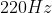, but a piano tuner hears 4 beats every 3 seconds when they are played together.  If one of the piano strings is vibrating correctly at, what must be the frequency of the other?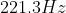only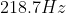onlyor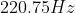onlyor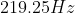orExplanation:

With the information provided we can determine the frequency of the beats.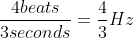This means that the string of the piano could either be this much higher or lower than thestring.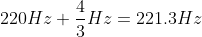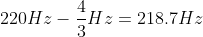### Example Question #986 : High School Physics

Two waves, each with an amplitude of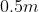are superimposed with constructive interference such that they are in phase. What is the resultant amplitude?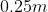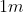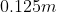Explanation:

When two waves are superimposed, the interference can either be constructive or destructive. In this case, the interference is constructive and the waves are in phase, which means we add the amplitudes together.

Since each wave has an amplitude of, our new amplitude will be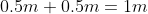.

### Example Question #1 : Interference And Superposition

Two identical waves are superimposed with destructive interference. What will happen to the resulting wave?

It will be twice as large as each original wave.

It will be half as large as each original wave.

The two waves will pass through each other without problem.

It will be still. There will be no wave.